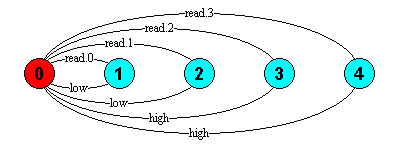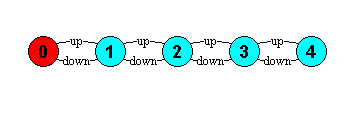Conditional

A conditional takes the form: if expr then local_process else local_process. FSP supports only integer expressions. A non-zero expression value causes the conditional to behave as the local process of the then part; a zero value causes it to behave as the local process of the else part. The else part is optional, if omitted and expr evaluates to zero the conditional becomes the STOP process.

Example:

```LEVEL = (read[x:0..3] ->
if x>=2 then
(high -> LEVEL)
else
(low -> LEVEL)).```Guards

A guarded transition takes the form (when B a -> P) which means that the action a is eligible when the guard B is true, otherwise a cannot be chosen for execution. The following example uses guards to define a bounded semaphore:

```const Max = 4
range Int = 0..Max

BSEMA(Init=0) = BSEMA[Init],
BSEMA[v:Int]  = (when (v<Max) up   -> BSEMA[v+1]
|when (v>0)   down -> BSEMA[v-1]
).
```